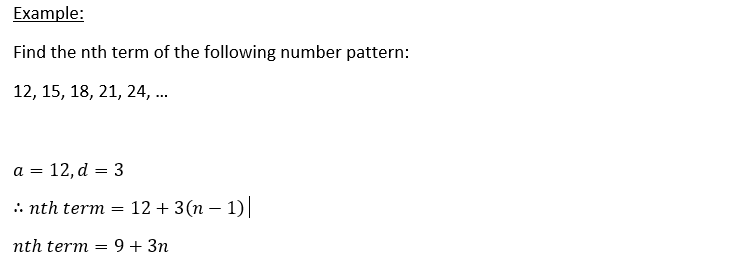• Mr Ausome

# O-Levels E-Math: Number Patterns and Sequences

Number Patterns is one of the chapters in O-level E-Math where students rarely have 100% confidence of getting it right during the exams. Most Singaporean students are pretty good at identifying patterns and have no problem spotting the logic behind each sequence. However, the difficulty often lies in coming up with a formula or an equation that expresses the Nth-term of the number sequence in terms of n.

Since every pattern is different, some students do not rely on formulas and they depend purely on their superior understanding to construct the nth term formula every time. However, this is relatively time consuming and the time spent is often not worth the marks allocated.

In this article, we will describe two of the more common patterns and introduce their general formulas such that there is a more structured and efficient way of deriving the nth-term formula.

Number Pattern Type 1: Constant Difference

Find the formula of the nth term of the sequence: 12, 15, 18, 21, 24, ...Notice that every term is 3 more than the previous term. This is the simplest kind of pattern where the increase/decrease in constant.

The General formula of this nth term for this number pattern sequence is:Number Pattern Type 2: Increasing Difference

Find the formula of the nth term of the sequence: 8, 13, 20, 29, 40, …Notice that the difference between the terms is increasing at a constant rate.

The General formula of this nth term for this number pattern sequence isPractice

Find the nth-term formula for the following sequences:

a) 5, 9, 13, 17, …

b) -7, -4, -1, 2, …

c) 6, 4, 2, 0, -2, …

d) 3, 5, 8, 12, 17, …

e) 0, 3, 8, 15, 24, …

a) nth term = 4n+1

b) nth term = 3n-10

c) nth term = -2n+8

d) nth term = 0.5 (n^2+n+4)

e) nth term = n^2 - 1

Hope this short tutorial on number patterns have helped you!

To get professional help (Math tuition) on secondary school mathematics in Singapore, click here.

Tags:

See All